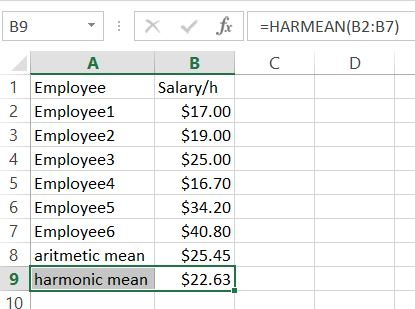#### How to Calculate Harmonic Mean in Excel

In this Excel tutorial you will teach yourself when to use harmonic mean and how to calulate it in Excel.

## When to use harmonic mean?

This average is used when data values are expressed in relative units, e.g. speed unit (miles/ h) or salary per hour of work (USD / h).

The use of the harmonic mean gives equal weight to each data. Using the arithmetic mean in this case would give more weight to the higher-valued data, and thus the mean would be overstated.

## How to calculate harmonic average in Excel?

The calculation method is to use the reciprocal of the sum of n data as the denominator and n as the numerator to compute the ratio. To calculate harmonic mean in Excel use HARMEAN function.

Syntax is very simple:

HARMEAN(number1, [number2], …)The formula I used is =HARMEAN(B2:B7)

As you may notice harmonic mean is much lower than aritmetic mean.

The following harmonic average formula can be used to express the relationship of the three averages:

weighted average >= geometric average >= harmonic mean

The harmonic mean is typically used when working with rates, such as speeds or growth rates, where values can be skewed by extreme outliers. In other cases, the arithmetic mean is a better representation of the central tendency of the data.

### Errors you may encounter

• #NUM! – The most likely reason is that one of your values is less than 0
• #VALUE! – one of the arguments is not a number. Check the data type